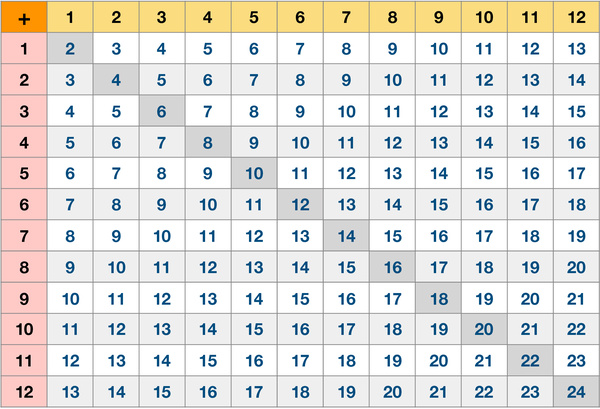# Addition Table 12 by 12Addition, subtraction, multiplication and division are the four elementary mathematical operations of arithmetic. Addition is the first operation kids learn. Around 6 years old, most kids are ready to learn facts about addition. Mastery of the small number addition facts is the foundation for learning other elementary arithmetic operations. There are many ways parents can work with kids to help them learn addition.

This worksheet provides a table of equations showing the sums from 1 + 1 up to 12 + 12. With the help of this table, parents could help kids learn, memorize, practice and recall the facts of adding small numbers easily. Once kids master this skill, they are well-prepared to tackle more advanced math topics such as multi-digit addition. It is also useful to explore patterns on the table to help kids memorize the facts.